﻿ Alternative Equations to Compute the Network and the Thermal Efficiency of the Irreversible Diesel Cycle Using Genetic Algorithm

### Alternative Equations to Compute the Network and the Thermal Efficiency of the Irreversible Diesel C...

Seyed Mahmood Mousavi, Javad Abolfazli Esfahani, Masoud Yazdi MamaghaniOPEN ACCESSPEER-REVIEWED

## Alternative Equations to Compute the Network and the Thermal Efficiency of the Irreversible Diesel Cycle Using Genetic Algorithm

Seyed Mahmood Mousavi1,, Javad Abolfazli Esfahani1, Masoud Yazdi Mamaghani1

### Abstract

In the current study, the effect of specific heat ratio, compression ratio, and the maximum to minimum temperature ratio of cycle on the thermal efficiency and the Network of irreversible Diesel cycle have been investigated. For this purpose, numerical solution and multi-objective genetic algorithms with a Pareto optimization method are used to determine the optimum values of specific heat ratio, compression ratio, and the maximum to minimum temperature ratio of cycle. The optimized values of the objective functions (namely Network and thermal efficiency) are then determined by using these vales. The numerical solution shows that the maximum Network and thermal efficiency is obtained simultaneously when the values of specific heat ratio, compression ratio, and the maximum to minimum temperature ratio of cycle are 1.3, 17.7, and 6.0, respectively, and these parameters are obtained by multi-objective genetic algorithms with a Pareto optimization method as 1.3, 17.57, and 6.0. The results obtained from current work are presented in the form of optimal equations for Network thermal efficiency in term of compression efficiency, expansion efficiency and compression ratio of the cycle. The results of current research work can provide a significant insight for optimal design of internal combustion engines including irreversibility.

### At a glance: Figures

123
Prev Next

• Mousavi, Seyed Mahmood, Javad Abolfazli Esfahani, and Masoud Yazdi Mamaghani. "Alternative Equations to Compute the Network and the Thermal Efficiency of the Irreversible Diesel Cycle Using Genetic Algorithm." American Journal of Mechanical Engineering 1.5 (2013): 119-125.
• Mousavi, S. M. , Esfahani, J. A. , & Mamaghani, M. Y. (2013). Alternative Equations to Compute the Network and the Thermal Efficiency of the Irreversible Diesel Cycle Using Genetic Algorithm. American Journal of Mechanical Engineering, 1(5), 119-125.
• Mousavi, Seyed Mahmood, Javad Abolfazli Esfahani, and Masoud Yazdi Mamaghani. "Alternative Equations to Compute the Network and the Thermal Efficiency of the Irreversible Diesel Cycle Using Genetic Algorithm." American Journal of Mechanical Engineering 1, no. 5 (2013): 119-125.

 Import into BibTeX Import into EndNote Import into RefMan Import into RefWorks

### 1. Introduction

Due to Diesel cycles features such as inherent fuel economy, easy operation, low maintenance, and long life, they have many applications in the field of transportation, marine, and stationary applications such as water pumping, power generation, and etc. Therefore, the optimization of Diesel cycle using different optimization techniques has been done by many researchers in recent years. Qin et al.  derived the universal relations between the network and compression ratio, the thermal efficiency and compression ratio, and the optimal relation between network and power efficiency. Moreover, they investigated the optimization of their model in order to examine the effect of cyclic processes on the performance of irreversible reciprocating heat engine cycle using numerical approach. Their results included the performance properties of irreversible reciprocating Diesel, Otto, Atkinson, and Brayton cycles. Ebrahmi  studied the performance optimization of specific heat ratio (SHR) of a Diesel cycle using detailed numerical approach. It was observed that SHR value of the working fluid had significant effects on the performance of the Diesel cycle, which should be considered in practical cycle analysis. Zheng and Lin  optimized the power output and the thermal efficiency of the heat engine cycle with respect to the pressure ratio. Yanlin et al.  investigated the optimal paths of piston motion of an irreversible Diesel cycle (IDC) to generate the minimum entropy. They found that optimizing the piston motion trajectories would result in reduction of engine entropy generation by more than 20%. Mathur et al.  optimized the compression ratio of Diesel fuelled variable compression ratio engine for variable load at a constant speed operation. They found that at rated speed of 1500 rpm, the compression ratio of 17 would show the highest brake thermal efficiency, and also the compression ratio of 18 exhibited marginally lower brake thermal efficiency, compared to the optimum compression ratio. Zhao et al.  studied the optimal performance of a Diesel heat engine taking the assumption of irreversibility due to the compression and expansion processes, finite rate heat transfer processes, and heat leak loss through the cylinder wall. They found the optimum network exist between the case of maximum efficiency and maximum network , while the optimum efficiency was higher than the efficiency at maximum network and lower than the maximum efficiency. Also they found that the optimal ranges of the network and efficiency decline with the decrease of the compression and expansion efficiencies. Zheng  investigated a unified cycle model of an internal combustion engine and obtained their optimum performance characteristics. Zheng’s results were similar to Zhao’s results .

However, in recent years, for optimization in engineering design, more and more attentions have been paid on multi-objective genetic algorithm (MOGA), which mimics the natural selection processing which a superior creature evolves whilst inferior creatures fade out from their population as generations go by Hiroasu et al. , Farhang-Mehr and Azarm  and Li . Hiroyasu et al.  optimized the Diesel engine emissions and fuel economy by using the NCGAs (neighborhood cultivation genetic algorithms) and phenomenological model. They used HIDECS (Hiroshima university Diesel engine combustion simulation) code which is based on the phenomenological model for the analysis of the Diesel engine. The amount of SFC, NOx and soot are minimized by changing the rate of fuel injection. The result of that simulation proved that NCGA can successfully derive the Pareto optimum solutions. Such Pareto optimum solutions are very helpful for design purposes, because optimization by the GAs using phenomenological models do not need a high calculation cost. Hiroyasu et al.  optimized the Diesel engine emissions and fuel efficiency with air swirl, EGR (exhaust gas recirculation), injection timing and multiple injections by using the Diesel engine computational model and NCGA. They found that the addition of EGR reduces the NOx emission. Gorji and Mozaffari  optimized the maximum network and minimum entropy generation of an irreversible thermal engine as a multi-objective, multi-modal mechanical problem, using the MSB (mutable smart bee) algorithm. They found that MSB algorithm is superior or equal to some other famous optimization methods such as self-adapting penalty function genetic algorithms and improved the particle swarm optimization for the multimodal problems.

In present work, the effect of the SHR, compression ratio, and the variation of the maximum to minimum temperature ratio (TR) of cycle on the thermal efficiency and network of IDC have been investigated for high temperature gradients. Thereafter, the numerical solution and the MOGA with a Pareto method are used to the determination of the optimum values of SHR, compression ratio and TR of cycle. By using the optimum values of these parameters, the optimum values of the thermal efficiency and network of IDC have been calculated.

### 2. Governing Equations

The p-V and T-s diagrams for an IDC are illustrated in Figure 1 (a-b), which consist of two adiabatic processes (compression or expansion), an isobaric heat addition process, and an isochoric heat rejection process, where the processes 1–2 and 3–4 are isentropic or reversible adiabatic, 1–2i and 3–4i are irreversible adiabatic, 2–3 is an isobaric heat addition process, and 4i–1 is an isochoric heat rejection process.

In Diesel cycle, the heat transfer to the cycle is occurred during the isobaric process and the heat transfer from the cycle takes place by the isochoric process. Therefore the equations of these processes along with net¬ work and thermal efficiency are as the followings: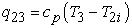(1)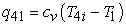(2)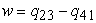(3)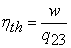(4)

Compression and expansion efficiencies may be used to describe the irreversibility of these processes. These equations are as the followings ;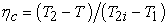(5)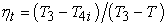(6)

Also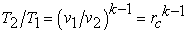(7)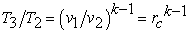(8)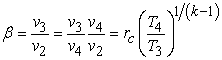(9)

From Eqs. (5-9) it can be concluded that: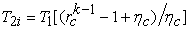(10)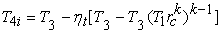(11)

where k=cp/cυ and rc =v1/v2. The work and isobaric heat addition can be formulated as: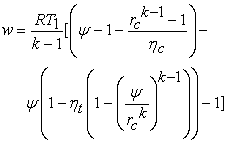(12)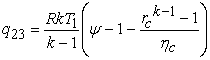(13)

where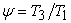(14)

From Eqs. (3-4, 12-14) the efficiency can be determined by: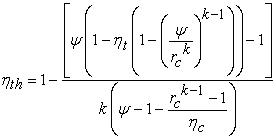(15)

As it is shown the efficiency is independent of the heat loss, although the heat loss was considered in Eq. (4).

### 3. Numerical Modeling

According to Refs. [Nature and Science, 7(9) 78-82. 2009.">14, Computers & Mathematics with Applications, 62 (5). 2169-2176. 2011.">15, 16, International Journal of Ambient Energy, 31 (2). 101-108. 2010.">17], and the above mentioned equations, which are used to calculate the net¬ work as well as the thermal efficiency, are solved using the following constants and range of parameters (R=0.287 kJ/kg.K, T1=298K, ηct=0.95, rc=5-30, ψ=5-6).

Figure 2a shows the distribution of network by variation of compression ratio and SHR at TR=5 while Figure 2b referred to TR=6. According to the Figure 2 (a-b), by increasing the compression ratio for all values of SHR in range of 1.3 to 1.41, the network increases initially and after reaching its maximum value, gradually reduced. Furthermore by considering Figure 2 (a-b), it is clear that in a constant compression ratio in range of 5 to 30 the SHR increases and network decreases continuously. By comparing Figure 2 (a-b), it’s important to note that the decrease of network is strongly associated with increasing SHR at fewer values of TR in the Diesel cycle. By attention to Figure 2a, the network value is negative when compression ratio and SHR is more than 26 and 1.4, respectively. It is evident from Figure 2 (a-b) that, by increasing TR the maximum network occurs at higher compression ratio, such a way that the maximum network obtains for compression ratio in range of 10 to 16 and 15 to 21 while TR is equal to 5 and 6 respectively. By increasing TR from 5 to 6, maximum network increase about 32%. This shows extreme dependency of network to TR of the cycle. Also minimum network value of the cycle is -40 kJ/kg and 160 kJ/kg when TR is equal to 5 and 6 respectively. It is worth noting that when SHR is equal to 1.3, the variation of network in low compression ratio is more compared to higher compression ratio values. By comparing Figure 2 (a-b), for all values of TR, the maximum network obtains while SHR is equal to 1.3. For low values of compression ratio (lower than 8), the network is nearly independent of SHR as the network is almost constant for all values of SHR.

Figure 3 shows the changes of network with the compression ratio for various SHR values and TR. As it is clear that, at a constant TR, the intensity of network variation increases by increasing SHR. As it is shown, the maximum network is obtained in lower compression ratio by increasing SHR. Such a way that, in TR and SHR are equal to 6 and 1.41 respectively, the maximum network is achieved while the compression ratio is equal to 9.2. It is obvious that the maximum network is obtained when the compression ratio, TR of cycle, and SHR are 18.3, 6, and 1.3, respectively.

Figure 4 (a-b) shows dependency of the thermal efficiency on the compression ratio and SHR at TR=5 and TR=6, respectively. According to Figure 4 (a-b), at constant SHR, when it is less than 1.36, for a TR, by increasing compression ratio, the thermal efficiency increases but when the SHR is more than 1.36, by increasing compression ratio, the thermal efficiency increases initially and after reaching its maximum value, gradually reduces, so that, the thermal efficiency is less than zero at low TRs. By comparing Figure 4a with Figure 4b, it is evident that, in a constant SHR, the thermal efficiency increases by increasing TR, such a way that the maximum thermal efficiency obtains for compression ratio in range of 12 to 14 and 16 to 19 while TR is identical with 5 and 6 respectively. By considering Figure 4 (a-b), it is clear that, at all values of TR, the maximum thermal efficiency is obtained when SHR is identical to 1.41.With respect to the Figure 4 (a-b), in a constant TR and compression ratio, the thermal efficiency decreases by decreasing SHR. Also the thermal efficiency is almost independent on SHR and it is constant in a SHR and compression ratio less than 8. An efficiency change drastically is more at higher TR. As it is clear, by comparing Figure 4 (a-b), by increasing TR, the efficiency is increased. By increasing TR, maximum efficiency is obtained at higher compression ratio.

Figure 5 shows the changes in the thermal efficiency with the compression ratio in different values of SHR and TR of cycle. The maximum thermal efficiency of IDC is located in the maximum values of TR of cycle and SHR. Meanwhile, when the compression ratiois lower than 10 and TR is equal to 5, the thermal efficiency is a little more than while the TR is equal to 6. As it is evident from Figure 5, the dependence of the thermal efficiency on the compression ratio in higher values of SHR is more sensible, so that when SHR is identical to 1.41, the variation of thermal efficiency is very high. Additionally this figure illustrates that the thermal efficiency is in its maximum value when the compression ratio, TR, and SHR are 16.20, 6, and 1.41, respectively.

Figure 6 shows the variation of thermal efficiency with the network in the same ranges of compression ratio, TR of cycle and SHR. As it is evident, in order to maximize the network and thermal efficiency simultaneously in any value of TR of cycle, the value of SHR should be 1.3. Also for any value of SHR, the maximum value of the network and thermal efficiency accrues when TR is equal to 6. It can be concluded that the dependence of network on SHR is higher compared to the thermal efficiency, meaning that at a particular value of TR of cycle, the effect of SHR is more dominant on the network of the cycle. If SHR is increased by 5%, the network is reduced up to 32%, but the thermal efficiency is increased up to 12%.

According to Figs. (2-5), the maximum value of network obtains for compression ratio in range of 15 to 21, TR and SHR are equal to 6 and 1.3, respectively. Also the maximum thermal efficiency acquires for compression ratio in range of 16 to 19, TR and SHR are equal to 6 and 1.41. Figure 7 shows the changes of maximum network and thermal efficiency by compression ratio changes. As it is evident that the optimum network and thermal efficiency obtains while the compression ratio is equivalent to 17.7.

### 4. Multi-objective Optimization

Multi-objective optimization, which is also called multi-criteria optimization or vector optimization, has been defined as finding a vector of decision variables satisfying constraints to give acceptable values to all objective functions . In general, it can be mathematically defined as:

Find the vector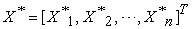to optimize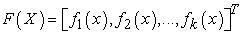(16)

Subject to m inequality constraints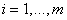(17)

and p equality constraints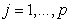(18)

where X* Rn is the vector of decision or design variables, and F(X) Rk is the vector of objective functions, which must each be either minimized or maximized. However, without the loss of generality, it is assumed that all objective functions are to be minimized. Such multi-objective minimization based on Pareto approach can be conducted using some definitions, which are presented here.

4.1. Definition of Pareto Dominance

A vector U = [u1, u2, . . . , uk] ∈ Rk is dominant to vector V = [v1, v2, . . . , vk] ∈ Rk (denoted by U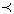V ) if and only if ∀i∈ {1, 2, . . . , k}, ui ≤ vi⊥∃j∈ {1, 2, . . . , k}: uj< vj. In other words, there is at least one uj which is smaller than vj whilst the remaining u’s are either smaller or equal to corresponding v’s.

4.2. Definition of Pareto Optimality

A point X* Ω (Ω is a feasible region in Rn satisfying Eqs. (17 and 18)) is said to be Pareto optimal (minimal) with respect to all X Ω if and only if F(X*)F(X). Alternatively, it can be readily restated as ∀i {1, 2, . . . , k}, X Ω - {X*}fi(X*) ≤ fi(X) ⊥∃j {1, 2, . . . , k}: fj(X*) < fj(X). In other words, the solution X* is said to be Pareto optimal (minimal) if no other solution can be found to dominate X* using the definition of Pareto dominance.

4.3. Definition of a Pareto Set

For a given multi-objective optimization problems, a Pareto set P* is a set in the decision variable space consisting of all the Pareto optimal vectors P*={XΩ |Ɇ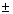X' Ω: F(X')F(X)}. In other words, there is no other X' as a vector of decision variables in Ω that dominates any X P*.

4.4. Definition of a Pareto Front

For a given multi-objective optimization problems, the Pareto front PF* is a set of vector of objective functions which are obtained using the vectors of decision variables in the Pareto set P*, that is PF* = {F(X) = (f1(X), f2(X), . . .fk(X)): X P*}. In other words, the Pareto front PF* is a set of the vectors of objective functions mapped from P*.

Evolutionary algorithms have been widely used for multi-objective optimization because of their natural properties suited for these types of problems. This is mostly because of their parallel or population-based search approach. Therefore, most of the difficulties and deficiencies within the classical methods in solving multi-objective optimization problems are eliminated. For example, there is no need for either several runs to find all individuals of the Pareto front or quantification of the importance of each objective using numerical weights . In this way, the original non-dominated sorting procedure given by Goldberg  was the catalyst for several different versions of multi-objective optimization algorithms . The optimization process of IDC can be formulated as.

### 5. Multi-objective Thermodynamic Optimization

5.1. Objective Functions

The aim of the optimization is determination of an optimal value for each of the parameters including SHR, compression ratio and the cycle’s TR. Afterwards by using the optimal values the best combination of thermal efficiency and network are obtained. In this context, two equations (12 and 15) which derived in section 2 are used as the objective functions.

5.2. Multi-objective Thermodynamic Optimization of an Irreversible Diesel Cycle

The behavior and performance of an IDC is dependent on different input and output parameters in a multi-objective optimal sense. The some input parameters involved in the thermodynamic analysis of the IDC are SHR (k=cp/cυ), compression ratio (rc12) and TR of cycle (ψ=T3/T1). The output parameters involved network (w) as well as the efficiency (ηth). According to Refs. [Nature and Science, 7(9) 78-82. 2009.">14, Computers & Mathematics with Applications, 62 (5). 2169-2176. 2011.">15, 16, International Journal of Ambient Energy, 31 (2). 101-108. 2010.">17] SHR (1.3<k<1.41), compression ratio (5<rc<30), and TR of cycle (5<ψ<6) are considered as design variables which must be optimally found based on multi-objective optimization of two output parameters, namely, network (w) as well as the thermal efficiency (ηth). Population size, crossover probability (Pc), and mutation probability (Pm) has been chosen 200, 0.6, and 0.3, respectively. The distribution of the solutions in Pareto-optimal front is shown in Figure 8. According to Figure 8 it can be seen that the optimization problem has been converged. The results of genetic algorithms show that in order to maximize the network and thermal efficiency simultaneously, the value of SHR, compression ratio, and TR of cycle must be 1.3, 17.57, and 6.0, respectively. As it is evident, the optimal MOGA values of SHR and TR are exactly equal to the values obtained by numerical solution of these values. The optimal MOGA value of compression ratio is different from numerical solution about 1.2%. A careful investigation of these Pareto optimization results reveals some interesting and informative design aspects.

In order to reduce the computational complexity of Network and thermal efficiency, according to the optimal values of SHR and TR of cycle, which are obtained by using numerical methods and genetic algorithms, the alternative optimal equations to calculate the network and the thermal efficiency are presented. The network equation is as follow: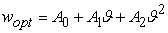(19)

where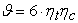(20)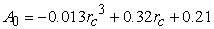(21)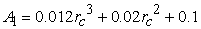(22)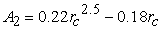(23)

And the optimal thermal efficiency equation is: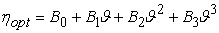(24)

where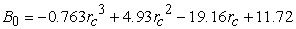(25)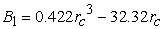(26)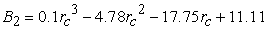(27)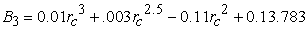(28)

Figure 9 (a-b) shows the comparison of the basic Eqs. (12) and (15) and alternative optimal Eqs. (19) and (24) of the networkand the thermal efficiency. According to Figure 9a, it is evident that, the results of the optimal equation with high accuracy (about 98%) are consistent with the basic equations. and the accuracy of the thermal efficiency optimal equation is about 99%. High accuracy of optimal equations is caused validity of these equations.

Figure 9. Variation of (a) Network and (b) thermal efficiency with variation of compression ratio

### 6. Conclusion

In this paper the effects of different parameters such as compression ratio, SHR and TR of IDC are investigated. The performance characteristics of the cycle are obtained by using a numerical approach. The results show that there are significant effects of the compression ratio, SHR, and TR on the performance of the cycle which should be considered in practical cycle analysis. The Pareto approach of MOGA is used for thermodynamic cycle optimization of the current cycle. The results obtained show that:

•  When SHR is equal to 1.3, the variation of network in low compression ratio is more than higher compression ratio values,

•  The dependency of network on SHR is more than thermal efficiency,

•  If SHR is increased by 5%, the network is reduced up to32%,

•  If SHR is increased by 5%, the thermal efficiency is increased up to 12%,

•  The maximum network is obtained when the compression ratio, SHR, and TR are equal to 18.3, 1.3 and 6, respectively,

•  The thermal efficiency is maximum when the compression ratio, SHR, and TR are 16.2, 1.41, and 6, respectively,

•  The numerical solution shows that the maximum network and thermal efficiency is obtained simultaneously when the values of SHR, compression ratio, and TR of cycle are 1.3, 17.7, and 6.0, respectively, and these parameters are obtained by MOGA as 1.3, 17.57, and 6.0,

•  The optimal MOGA values of SHR and TR are exactly equal to the values obtained by numerical solution, while the optimal MOGA values of compression ratio are different from the numerical solution about 1.2%.

•  The accuracy of the alternative optimal equations of the network and thermal efficiency are 98% and 99%, respectively.

It is worth noting that the results obtained in this paper are general and can provide some significant guidance for the optimal parameter design of heat engines.

### Nomenclature

cp ,cv　　　Specific heat capacity at constant pressure and volume

k　　　　　Specific heat ratio

Pc　　　　  Crossover probability

Pm　　　　 Mutation probability

q41　　　　Isochoric heat rejection

R　　　　   Universal gas constant

T　　　　   Absolute temperature

rc　　　　  Compression ratio

v　　　　   Volume

w　　　　   Network

### Greek Symbols

Β　　　　   Cutoff ratio

ηth　　　　Thermal efficiency

ηc, ηt　　　Compression and expansion efficiency

### Subscripts

I　　　　　Irreversibility

### References

  Qin, X., Chen, L., Sun, F., Wu, C, "The universal power and efficiency characteristics for irreversible reciprocating heat engine cycles," European journal of physics, 24 (4). 359-366. 2003.In article CrossRef  Ebrahimi, R., "Performance optimization of a Diesel cycle with specific heat ratio," Journal of American Science, 5 (8). 59-63. 2009.In article  Zheng, S., Lin, G., "Optimization of power and efficiency for an irreversible Diesel heat engine," Frontiers of Energy and Power Engineering in China, 4 (4). 560-565. 2010.In article CrossRef  Yanlin, G., Chen, L., Sun, F., "optimal paths of piston motion of irreversible diesel cycle for minimum entropy generation," Thermal science, 15 (4). 975-993. 2011.In article  Mathur, Y.B., Poonia, M.P., Jethoo, A.S., Singh, R., "Optimization of Compression Ratio of Diesel Fuelled Variable Compression Ratio Engine," International Journal of Energy Engineering (IJEE), 2 (3). 99-101. 2012.In article  Zhao, Y., Lin, B., Zhang, Y., Chen, J., "Performance analysis and parametric optimum design of an irreversible Diesel heat engine," Energy Conversion and Management, 47 (18-19). 3383-3392. 2006.In article CrossRef  Zheng, S., "Unified cycle model of a class of internal combustion engines and their optimum performance characteristics," Frontiers in Energy, 5 (4). 367-375. 2011.In article  Hiroyasu, T., Miki, M., Kamiura, J., Watanabe, S., "Multi-Objective Optimization of Diesel Engine Emissions and Fuel Economy using Genetic Algorithms and Phenomenological Model," Society of Automotive Engineers, 183. 1-12. 2002.In article  Farhang-Mehr, A., Azarm, S, "Entropy-based multi-objective genetic algorithm for design optimization," Structural and Multidisciplinary Optimization, 24 (5). 351-361. 2002.In article CrossRef  Li, J., "Multiobject optimization of a centrifugal impeller using evolutionary algorithms," Chinese Journal of Mechanical Engineering, 17 (3). 389-393. 2004.In article CrossRef  Hiroyasu, T., Miki, M., Kamiura, J., Watanabe, S., "Genetic Algorithms Optimization of Diesel Engine Emissions and Fuel Efficiency with Air Swirl, EGR, Injection Timing and Multiple Injections", Society of Automotive Engineers of Japan, Inc., 2003.In article CrossRef PubMed  Gorji-Bandpy, M., Mozaffari, A., "Multiobjective optimization of irreversible thermal engine using mutable smart bee algorithm," Applied Computational Intelligence and Soft Computing, 2012. 5-5.2012.In article  Gupta, H.N., Fundamentals of internal combustion engines, Prentice Hall of India, New Delhi, 2006.In article  Ebrahimi, R., "Thermodynamic modeling of performance of an irreversible diesel cycle with engine speed," Nature and Science, 7(9) 78-82. 2009.In article  Ebrahimi, R., "Thermodynamic modeling of performance of a Miller cycle with engine speed and variable specific heat ratio of working fluid," Computers & Mathematics with Applications, 62 (5). 2169-2176. 2011.In article CrossRef  Heywood, J.B., Internal combustion engine fundamentals, McGraw-Hill, New York, 1988.In article PubMed  Ebrahimi, R., Chen, L. "Effects of variable specific heat ratio of working fluid on the performance of an irreversible Diesel cycle," International Journal of Ambient Energy, 31 (2). 101-108. 2010.In article CrossRef  Coello C.A., Christiansen, A.D., "Multi-objective optimization of trusses using genetic algorithms," Computers & Structures, 75 (6). 647-660. 2000.In article CrossRef  Atashkari, K., Nariman-Zadeh, N., Pilechi, A., Jamali, A., Yao, X., "Thermodynamic Pareto optimization of turbojet engines using multi-objective genetic algorithms," International Journal of Thermal Sciences, 44 (11). 1061-1071. 2005.In article CrossRef  Goldberg, D.E., Genetic algorithms in search, optimization, and machine learning, Addison-Wesley Pub. Co., Reading, Mass., 1989.In article  Srinivas, N., Kalyanmoy, N., "Multi-objective Optimization Using Nondominated Sorting in Genetic Algorithms," Evolutionary Computation, 2 (3). 221-248. 1994.In article